This topic is locked

# Guide 2 - For PHPRunner Newbies - Export Excel

9/14/2020 6:57:28 AM
F
fhumanes authorThis time we are going to see a method to obtain Excel sheets more advanced than the exports that PHPRunner offers us.
Objective
The example, very simple, but which I understand can offer the basis for other more complex needs, is generated by the requirement of producing an Excel sheet of "Master" and "Detail" data from a data set.
Data
The example data model is: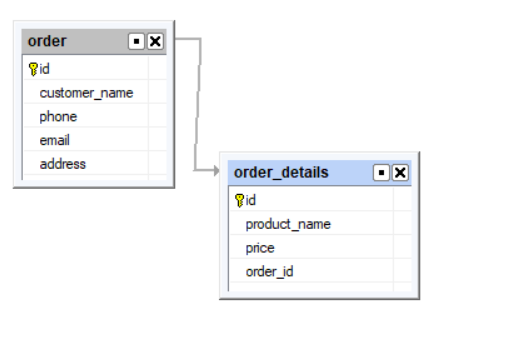As we can see, we have the sales "Orders" and depending on these, we have the "Order Detail" with their items and prices.
Result.
What we want to obtain, in this case, is an Excel result of this type: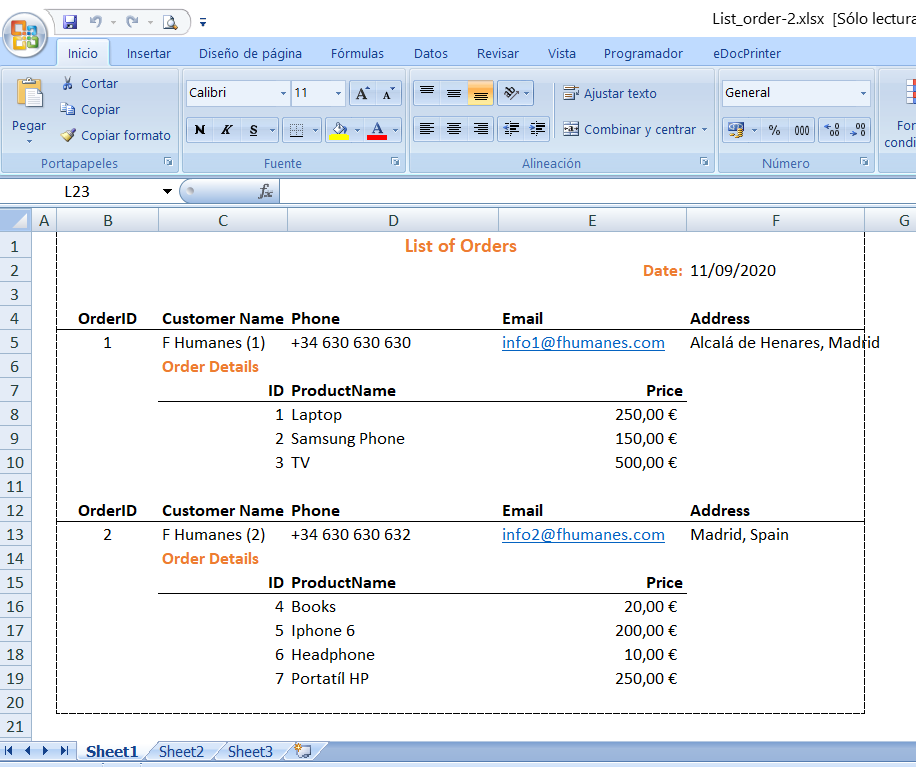DEMO: https://fhumanes.com/novel2/
Solution
As in other examples that I have in my portal, I oriented the solution to use the PHP library of PHPoffice/phpspreadsheet, which is the evolution of PHPExcel, this is the PHP library that PHPRunner brings to create exports to Excel.
To simplify the preparation, I use an Excel file template that has 3 sheets, the same as seen in the solution.
The information is distributed as follows:

• Sheet1. It is where the data and presentation required by the user will be obtained.
• Sheet2. It is where the PHP program will store the data set retrieved from the database.
• Sheet3. Where we are going to define the presentation of the data. This simplifies programming and also any Excel user could modify the template, and therefore, the presentation of the result without having to change anything in the programming.

The template looks like this: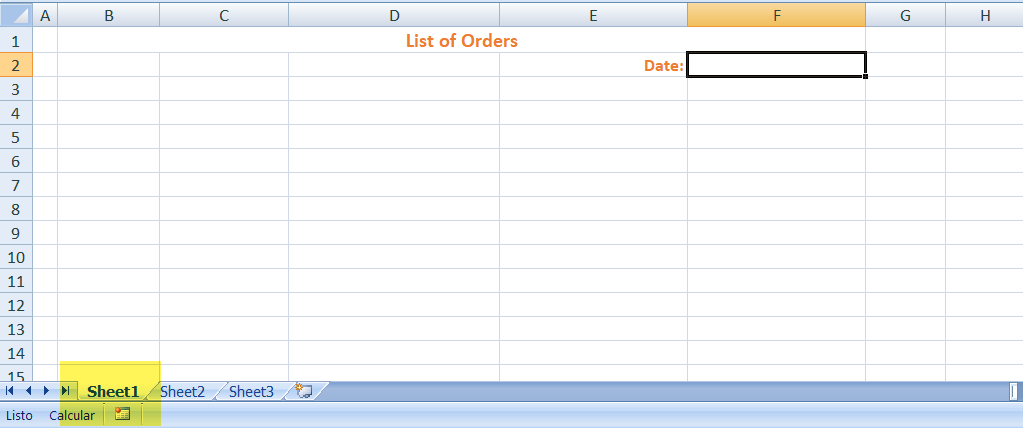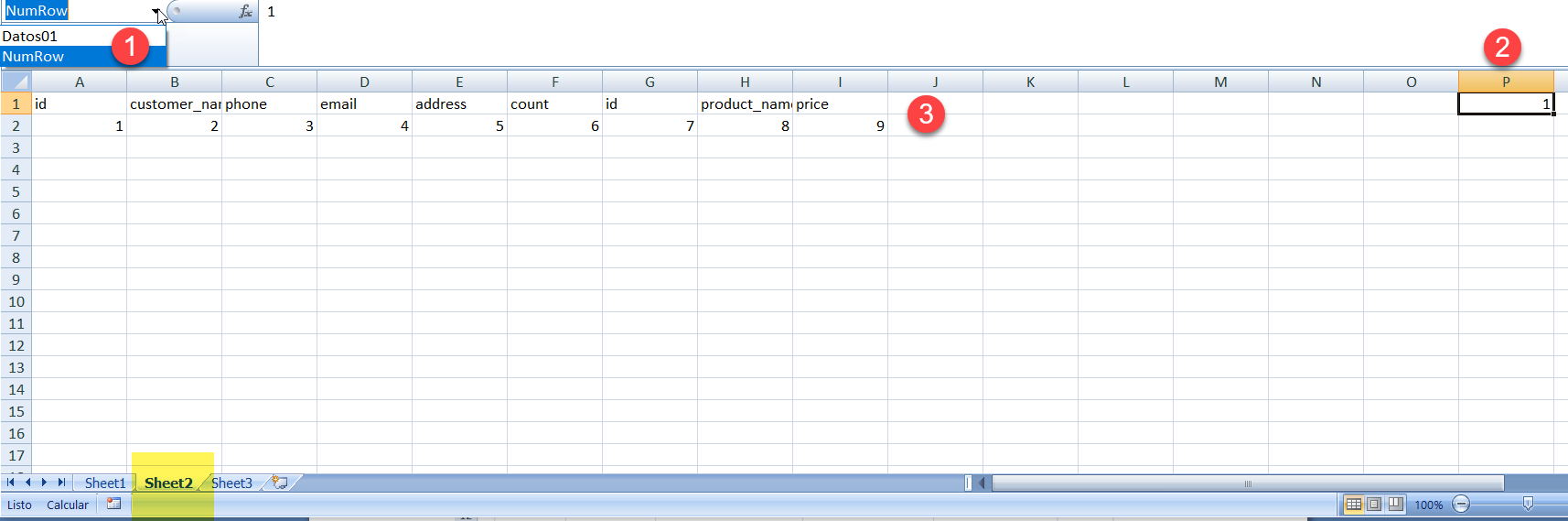(1) We use 2 "Names" (definition of cell ranges) to:

• Data01.- Define the table of the data set that we retrieve from the database.
• NumRow.- For all the formulas that we use in the 3rd sheet (presentation and calculations), it will indicate an index on the data table.

(2) Represents the name "NumRow". It can be put anywhere in the notebook.
(3) Represents the name "Data01" and must start at the point where it is. The number of fields to retrieve can grow as long as it is needed, but we must take it into account in the PHP coding that we will see later.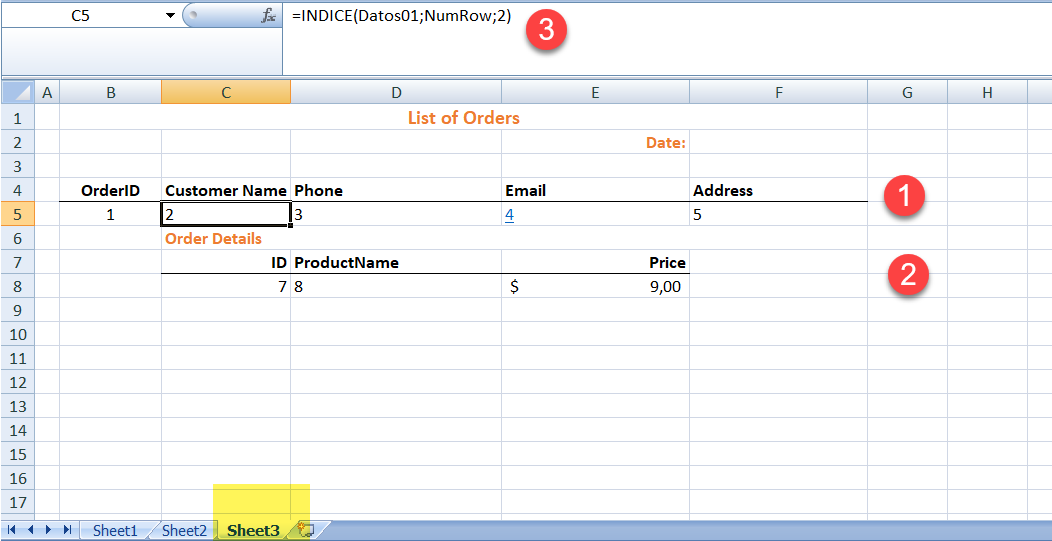(1) It is the presentation of the data from the "Master" table.
(2) It is the representation of the data in the "Detail" table.
(3) In all the cells that we use the recovered data, we use this formula (which is in Spanish in the image). As we can see, it refers to the name of the range of the table of the retrieved data, then it indicates the record number of the table and finally the column number of the table.
Once we have the template, we only have to write the PHP code that must be executed, either by means of a button or as in the case of the example, a menu option that makes the report.
To facilitate coding I have created 2 functions:

`// Function for copy row of sheet of Excelfunction copy_row_excel(int \$num_page_orig, int \$num_page_dest, int \$num_row_orig, int \$num_row_dest, int \$count_row, int \$limit_colum )`

We will use this function to copy the cells from "Sheet3" to "Sheet1". Contents and formats or styles are copied.

`// Assign value to reference of formulasfunction reference_data(int \$num_data, int \$num_row, int \$count_row, int \$limit_colum )`

This function substitutes the literal "NumRow" in the formulas for the row of the corresponding data table.
I show the complete code of the report creation

`<?php// Required by PHPRunner (security)@ini_set("display_errors","1");@ini_set("display_startup_errors","1");require_once("../../include/dbcommon.php");// Load the excel sheet management library classesrequire_once __DIR__ . '/../../../ComponentCode/PhpSpreadsheet_1.10/autoload.php'; use PhpOffice\PhpSpreadsheet\IOFactory;use PhpOffice\PhpSpreadsheet\Shared\Date;use PhpOffice\PhpSpreadsheet\Spreadsheet;use PhpOffice\PhpSpreadsheet\Writer\Xlsx;use PhpOffice\PhpSpreadsheet\NamedRange;// Template processor instance creation// \$reader = IOFactory::createReader('Xlsx');\$reader = new \PhpOffice\PhpSpreadsheet\Reader\Xlsx();// Options for reading excel files// \$reader->setReadDataOnly(true);\$reader->setIncludeCharts(true);// \$reader->setReadFilter();// \$reader->setReadEmptyCells(true);\$spreadsheet = \$reader->load(__DIR__ . '/template1.xlsx'); // read template\$spreadsheet->setActiveSheetIndex(0); // Activate the first sheet// Capture data from the BD\$sql="SELECTo.id id,o.customer_name,o.phone,o.email,o.address,countod,od.id idod,od.product_name,od.priceFROM `order` ojoin order_details od on (o.id = od.order_id)join ( select order_id, count(*) countod from order_details group by order_id ) T1 on (o.id = T1.order_id)order by o.id, od.id";\$result = \$conn->query(\$sql);\$data = \$result->fetch_all(MYSQLI_NUM); // faster - All record\$dataRows = count(\$data);// head\$DateList=now();\$spreadsheet->getActiveSheet()->setCellValue('F2' . '', Date::PHPToExcel(\$DateList)); // Fill in a cell with the current date in excel format\$spreadsheet->setActiveSheetIndex(1); // Activate the second sheet\$spreadsheet->getActiveSheet()->fromArray(\$data, null, 'A2'); // Load ALL records in the active sheet// Define named ranges\$spreadsheet->addNamedRange(new NamedRange('Datos01', \$spreadsheet->getActiveSheet(), 'A2:'.'I'.(\$dataRows-1+2)));\$master = NULL;\$num_row = 3; // Firt line of Reportfor (\$x = 0; \$x < \$dataRows; \$x++) { // All Record if (\$master <> \$data[\$x]) { // Change of Master \$master = \$data[\$x]; // New Master // Copy template of page 3 to page 1 copy_row_excel(2, 0, 3, \$num_row, 7, 6 ); \$spreadsheet->setActiveSheetIndex(0); // Activate the first sheet // \$spreadsheet->getActiveSheet()->insertNewRowBefore(\$num_row+6, (\$data[\$x])); // Copy presentation formats reference_data(\$x, \$num_row+2, 1, 6); // Head of Order \$num_row = \$num_row + 5; } copy_row_excel(2, 0, 8, \$num_row, 1, 6 ); reference_data(\$x, \$num_row, 1, 6); // for each record \$num_row = \$num_row + 1;}\$spreadsheet->addNamedRange(new NamedRange('DatosPrint001', \$spreadsheet->getActiveSheet(), 'A4:'.'F'.(\$num_row))); // Define print range// Show/hide gridlines when printing\$spreadsheet->getActiveSheet()->setShowGridlines(false); // Disable grid in print// Config print file Excel// \$spreadsheet->getActiveSheet()->getPageSetup()->setOrientation(\PhpOffice\PhpSpreadsheet\Worksheet\PageSetup::ORIENTATION_LANDSCAPE);\$spreadsheet->getActiveSheet()->getPageSetup()->setOrientation(\PhpOffice\PhpSpreadsheet\Worksheet\PageSetup::ORIENTATION_PORTRAIT);\$spreadsheet->getActiveSheet()->getPageSetup()->setPaperSize(\PhpOffice\PhpSpreadsheet\Worksheet\PageSetup::PAPERSIZE_A4);// Page Setup: Scaling options\$spreadsheet->getActiveSheet()->getPageSetup()->setFitToPage(false);\$spreadsheet->getActiveSheet()->getPageSetup()->setScale(90);// \$spreadsheet->getActiveSheet()->getPageSetup()->setFitToWidth(0); // \$spreadsheet->getActiveSheet()->getPageSetup()->setFitToHeight(0);// Page margins// \$spreadsheet->getActiveSheet()->getPageMargins()->setTop(1);// \$spreadsheet->getActiveSheet()->getPageMargins()->setRight(0.6);// \$spreadsheet->getActiveSheet()->getPageMargins()->setLeft(0.6);// \$spreadsheet->getActiveSheet()->getPageMargins()->setBottom(1);// Center a page horizontally/vertically\$spreadsheet->getActiveSheet()->getPageSetup()->setHorizontalCentered(false);\$spreadsheet->getActiveSheet()->getPageSetup()->setVerticalCentered(false);// Setting the print header and footer of a worksheet// \$spreadsheet->getActiveSheet()->getHeaderFooter()->setOddHeader('&C&HPlease treat this document as confidential!');\$spreadsheet->getActiveSheet()->getHeaderFooter()->setOddFooter('&L&B' . \$spreadsheet->getProperties()->getTitle() . '&RPage &P of &N');// Setting rows/columns to repeat at top/left\$spreadsheet->getActiveSheet()->getPageSetup()->setRowsToRepeatAtTopByStartAndEnd(1, 2);// Specify printing area\$spreadsheet->getActiveSheet()->getPageSetup()->setPrintArea('B1:'.'F'.(\$num_row));// Create file XLSX// Set active sheet index to the first sheet, so Excel opens this as the first sheet\$spreadsheet->setActiveSheetIndex(0);// Redirect output to a client's web browser (Xlsx)// header('Content-Type: application/vnd.openxmlformats-officedocument.spreadsheetml.sheet');// header('Content-Disposition: attachment;filename="municipios.xlsx"');// header('Cache-Control: max-age=0');// If you're serving to IE 9, then the following may be needed// header('Cache-Control: max-age=1');// If you're serving to IE over SSL, then the following may be neededheader('Expires: Mon, 26 Jul 1997 05:00:00 GMT'); // Date in the pastheader('Last-Modified: ' . gmdate('D, d M Y H:i:s') . ' GMT'); // always modifiedheader('Cache-Control: cache, must-revalidate'); // HTTP/1.1header('Pragma: public'); // HTTP/1.0// Old system based on a temporary file// -------------------- v foot to save the new Excel document ------------------\$temp_file = tempnam(sys_get_temp_dir(), 'Excel');// Save EXCEL// \$writer = new Xlsx(\$spreadsheet);\$writer = IOFactory::createWriter(\$spreadsheet, 'Xlsx');\$writer->setPreCalculateFormulas(false); // Disable formula validation - to improve response time.\$writer->save(\$temp_file);// ------------------ Operation with file result -------------------------------------------\$documento = file_get_contents(\$temp_file);unlink(\$temp_file); // delete file tmpheader("Content-Disposition: attachment; filename= List_order.xlsx");header('Content-Type: application/excel');echo \$documento;// Alternative method, but also creates a temporary// \$writer = IOFactory::createWriter(\$spreadsheet, 'Xlsx');// \$writer->save('php://output');// ----------------------------------- Functions ------------------------// Function for copy row of sheet of Excelfunction copy_row_excel(int \$num_page_orig, int \$num_page_dest, int \$num_row_orig, int \$num_row_dest, int \$count_row, int \$limit_colum ) { global \$spreadsheet; \$values = array(); \$styles = array(); for (\$a= 0; \$a < \$count_row; \$a++){ \$spreadsheet->setActiveSheetIndex(\$num_page_orig); // Activate the sheet for (\$b= 0; \$b < \$limit_colum; \$b++){ // Copy de Values \$ref=chr((ord('A')+\$<img src='https://asprunner.com/forums/public/style_emoticons/<#EMO_DIR#>/cool.gif' class='bbc_emoticon' alt='B)' />).(\$num_row_orig+\$a); // Origen \$values[\$a][\$b] = \$spreadsheet->getActiveSheet()->getCell(\$ref)->getValue(); // Value \$style = \$spreadsheet->getActiveSheet()->getStyle(\$ref); // Style \$style = serialize(\$style); // Style \$styles[\$a][\$b] = \$style; // Style } } for (\$a= 0; \$a < \$count_row; \$a++){ \$spreadsheet->setActiveSheetIndex(\$num_page_dest); // Activate the sheet for (\$b= 0; \$b < \$limit_colum; \$b++){ // Copy de Values \$ref=chr((ord('A')+\$<img src='https://asprunner.com/forums/public/style_emoticons/<#EMO_DIR#>/cool.gif' class='bbc_emoticon' alt='B)' />).(\$num_row_dest+\$a); // Origen \$spreadsheet->getActiveSheet()->setCellValue(\$ref,\$values[\$a][\$b]); // Value \$style = unserialize(\$styles[\$a][\$b]); // Style \$spreadsheet->getActiveSheet()->duplicateStyle(\$style,\$ref); // Style } } return true;}// Assign value to reference of formulasfunction reference_data(int \$num_data, int \$num_row, int \$count_row, int \$limit_colum ) { global \$spreadsheet; global \$data; \$values = 0; \$spreadsheet->setActiveSheetIndex(0); // Activate the sheet for (\$a= 0; \$a < \$count_row; \$a++){ for (\$b= 0; \$b < \$limit_colum; \$b++){ // Copy de Values \$ref=chr((ord('A')+\$<img src='https://asprunner.com/forums/public/style_emoticons/<#EMO_DIR#>/cool.gif' class='bbc_emoticon' alt='B)' />).(\$num_row+\$a); \$values = \$spreadsheet->getActiveSheet()->getCell(\$ref)->getValue(); // Value \$values = str_replace("NumRow", \$num_data+1 , \$values); switch (\$<img src='https://asprunner.com/forums/public/style_emoticons/<#EMO_DIR#>/cool.gif' class='bbc_emoticon' alt='B)' /> { case 2: // \$spreadsheet->getActiveSheet()->setCellValue(\$ref1,'=IF(U'.(6+\$<img src='https://asprunner.com/forums/public/style_emoticons/<#EMO_DIR#>/cool.gif' class='bbc_emoticon' alt='B)' />.'<>U'.(7+\$<img src='https://asprunner.com/forums/public/style_emoticons/<#EMO_DIR#>/cool.gif' class='bbc_emoticon' alt='B)' />.',1,B'.(6+\$<img src='https://asprunner.com/forums/public/style_emoticons/<#EMO_DIR#>/cool.gif' class='bbc_emoticon' alt='B)' />.'+1)'); // Valor // break; default: \$spreadsheet->getActiveSheet()->setCellValue(\$ref,\$values); // Valor } } } return true;}?>`

In my portal you can have all the files you need to deploy the example on your computers. Although the example is in version 10.4 of PHPrunner, this encoding would be valid for any version, as long as the PHP is version 7.X
For any questions or clarifications, please, write me an email to [email="fernandohumanes@gmail.com"]fernandohumanes@gmail.com[/email]